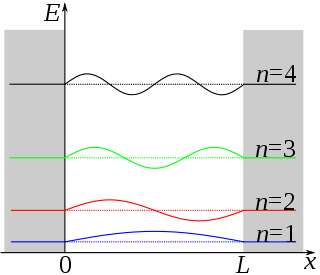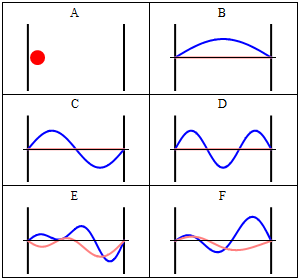Lecture 8: Topical Overview of PIB and Postulates QM

•• Contributed by Delmar Larsen
• Founder and Director at Libretexts

Overview of Lecture 7:

Wavefunctions have a probabilistic interpretation. More specifically, the wavefunction squared (or to be more exact, the $$\Psi^*\Psi$$ is a probability density). To get a probability, we have to integrate $$\Psi^*\Psi$$ over an interval. We can never discuss the probability at one point, except in a relative fashion or if we are referring to a node (where it will never be found). The probabilistic interpretation comes with key features of $$\Psi^*\Psi$$ including finite, nonnegative and not infinite. A key aspect of thisis that the wavefunctions must be normalized.

We the introduced the particle in the box, which is arguable the mode important "trapped" particle system. This system is "easy" to solve the Schrödinger Equation to get oscillatory wavefunctions.

The Particle in a one Dimensional box (a Trapped Particle)

The particle in the box model system is the simplest non-trivial application of the Schrödinger equation, but one which illustrates many of the fundamental concepts of quantum mechanics. For a particle moving in one dimension (again along the x- axis), the Schrödinger equation can be written

$-\dfrac{\hbar^2}{2m}\psi {}''(x)+ V (x)\psi (x) = E \psi (x)\label{3.5.1}$

Assume that the particle can move freely between two endpoints x = 0 and x = L, but cannot penetrate past either end. This is equivalent to a potential energy dependent on x with

$V(x)=\begin{cases} 0 & 0\leq x\leq L \\ \infty & x< 0 \; and\; x> L \end{cases}\label{3.5.2}$

This potential is represented by the dark lines in Figure $$\ref{3.5.1}$$. The infinite potential energy constitutes an impenetrable barrier since the particle would have an infinite potential energy if found there, which is clearly impossible.The barriers outside a one-dimensional box have infinitely large potential, while the interior of the box has a constant, zero potential.

The particle in a one dimensional box is not a real system.

As seen before with vibrating strings in the classical wave function, $$X(x) = A\sin(kx)\psi (x)$$. In this system,

$\color{red} {\psi (x) = B\sin \left(\dfrac{n\pi x}{L}\right)} \label{1a}$

for

$0 \leq x \leq L \label{1b}$

and

$$\psi (x) = 0$$ for $$x < 0$$ and $$x > L$$.

This results in the quantization in levels. For the one dimensional box, the eigenvalue is

$\color{red} {E_n = \dfrac{h^2 n^2 }{8m_e a^2}}$

These are the only values of the energy which allows solutions of the Schrö​dinger Equation $$\ref{3.5.1}$$ consistent with the boundary conditions in Equation $$\ref{3.5.4}$$. The integer $$n$$, called a quantum number, is appended as a subscript on $$E$$ to label the allowed energy levels. Negative values of $$n$$ add nothing new because the energies in Equation $$\ref{3.5.11}$$ depends on $$n^2$$.Figure $$\PageIndex{2}$$: A plot of $$ψ_n(x)$$ for the first four wavefunctions.

Transition from one Eigenstate to another

Like the hydrogen atom, you can figure out the spectrum for transitions from $$n_1$$ to $$n_2$$ by $$\delta E = E_{n_2} - E_{n_1} = hc/\lambda$$.

Normalize the Wavefunction

To normalize the wave function $$\psi (x)$$ it is important to recognize that $$\int_{-\infty}^{\infty} \psi_n^* \psi_n dx$$ is the probability of finding a particle in all space, which is 1, or 100%. The integral can be broken up into three parts, defined by the system's boundary conditions:

$\int_{-\infty}^{0} \psi_n^* \psi_n dx+ \int_{0}^{L} \psi_n^* \psi_n dx + \int_{a}^{\infty} \psi_n^* \psi_n dx = 1 \label{PIB2}$

since $$\psi (x) = 0$$ when $$x<0$$ and $$x>a$$, the first and last integral of the summation of integrals are zero. That leaves $$N^2\int_{0}^{L} \psi_n^* \psi_n dx\ = 1$$, where $$N$$ is the normalization constant. Thus,

$1=N^2 \int_0^a {\sin}^2(n\pi x/L)dx = N^2(L/2) \label{PIB3}.$

Solving for $$N$$ = $$\sqrt{2/a}$$. Going back to $$\psi (x) = B\sin(\frac {n\pi x}{a})$$, the new equation becomes

$\color{red} {\psi (x) = \sqrt{\frac{2}{a}}\sin(\dfrac{n\pi x}{L})} \label{PIB4}$Some trajectories of a particle in a box according to Newton's laws of classical mechanics (A), and according to the Schrödinger equation of quantum mechanics (B-F). In (B-F), the horizontal axis is position, and the vertical axis is the real part (blue) and imaginary part (red) of the wavefunction. The states (B,C,D) are energy eigenstates, but (E,F) are not.

Java simulation of particles in boxes :https://phet.colorado.edu/en/simulation/bound-states

Normalization of the Wavefunction

Since wavefunctions can in general be complex functions, the physical significance cannot be found from the function itself because the $$\sqrt {-1}$$ is not a property of the physical world. Rather, the physical significance is found in the product of the wavefunction and its complex conjugate, i.e. the absolute square of the wavefunction, which also is called the square of the modulus.

$\psi^*(r , t ) \psi (r , t ) = {|\psi (r , t)|}^2 \label {3.6.1}$

where $$r$$ is a vector (x, y, z) specifying a point in three-dimensional space. The square is used, rather than the modulus itself, just like the intensity of a light wave depends on the square of the electric field.

probability is a real number between 0 and 1. An outcome of a measurement which has a probability 0 is an impossible outcome, whereas an outcome which has a probability 1 is a certain outcome. According to Equation $$\ref{3.6.1}$$, the probability of a measurement of $$x$$ yielding a result between $$-\infty$$ and $$+\infty$$ is

$P_{x \in -\infty:\infty}(t) = \int_{-\infty}^{\infty}\vert\psi(x,t)\vert^{ 2} dx. \label{3.6.2}$

However, a measurement of $$x$$ must yield a value between $$-\infty$$ and $$+\infty$$, since the particle has to be located somewhere. It follows that $$P_{x \in -\infty:\infty}(t) =1$$, or

$\int_{-\infty}^{\infty}\vert\psi(x,t)\vert^{ 2} dx = 1, \label{3.6.3}$

which is generally known as the normalization condition for the wavefunction.

Example $$\PageIndex{1}$$: Normalizing a Gaussian wavepacket

Normalize the wavefunction of a Gaussian wave packet, centered on $$x=x_o$$, and of characteristic width $$\sigma$$: i.e.,

$\psi(x) = \psi_0 {\rm e}^{-(x-x_0)^{ 2}/(4 \sigma^2)}. \label{3.6.4}$

To determine the normalization constant $$\psi_0$$, we simply substitute Equation $$\ref{3.6.4}$$ into Equation $$\ref{3.6.3}$$, to obtain

$\vert\psi_0\vert^{ 2}\int_{-\infty}^{\infty}{\rm e}^{-(x-x_0)^{ 2}/(2 \sigma^2)} dx = 1. \label{3.6.5}$

Changing the variable of integration to $$y=(x-x_0)/(\sqrt{2} \sigma)$$, we get

$\vert\psi_0\vert^{ 2}\sqrt{2} \sigma \int_{-\infty}^{\infty}{\rm e}^{-y^2} dy=1. \label{3.6.6}$

However,

$\int_{-\infty}^{\infty}{\rm e}^{-y^2} dy = \sqrt{\pi}, \label{3.6.7}$

which implies that

$\vert\psi_0\vert^{ 2} = \dfrac{1}{(2\pi \sigma^2)^{1/2}}. \label{3.6.8}$

Hence, a general normalized Gaussian wavefunction takes the form

$\psi(x) = \dfrac{e^{\rm{i} \phi}}{(2\pi \sigma^2)^{1/4}}e^{-(x-x_0)^2/(4 \sigma^2)} \label{3.6.9}$

where $$\phi$$ is an arbitrary real phase-angle.s

Example $$\PageIndex{2}$$: Normalizing the Eigenstates of the particle in a box

To normalize the wave function $$\psi (x)$$ it is important to recognize that $$\int_{-\infty}^{\infty} \psi_n^* \psi_n dx$$ is the probability of finding a particle in all space, which is 1, or 100%.

The integral can be broken up into three parts, defined by the system's boundary conditions:

$\int_{-\infty}^{0} \psi_n^* \psi_n dx+ \int_{0}^{a} \psi_n^* \psi_n dx + \int_{a}^{\infty} \psi_n^* \psi_n dx = 1$

since $$\psi (x) = 0$$ when $$x<0$$ and $$x>a$$, the first and last integral of the summation of integrals are zero. That leaves

$N^2\int_{0}^{a} \psi_n^* \psi_n dx\ = 1$

where $$N$$ is the normalization constant. Thus,

$1=N^2 \int_0^a {\sin}^2(n\pi x/a)dx = N^2(a/2) \label{3}$.

Solving for $$N$$ = $$\sqrt{2/a}$$. Going back to $$\psi (x) = B\sin(\frac {n\pi x}{a})$$, the new equation becomes

$\psi (x) = \sqrt{\frac{2}{a}}\sin(\frac{n\pi x}{a}) \label{4}$

Now back to the simulation... to get some intuition.

Java simulation of particles in boxes :https://phet.colorado.edu/en/simulation/bound-states